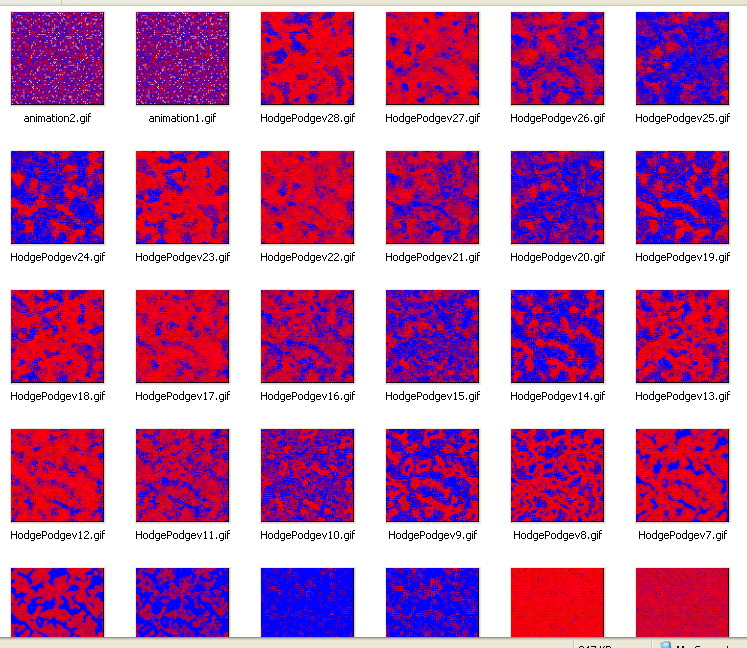The HodgePodge Cellular AutomatonThis implements in Liberty Basic the cellular automaton I first met published in August 1988 Scientific American, in an A K Dewdney article. This was at the time when the game of Life was also being popularised.
In a grid (here I use 100x100) each cell takes values from 0 ( 'uninfected') to say 100 ( 'ill')
Each cell has a 'neighbourhood' of either four orthogonally ( Von Neumann) , or 8, including diagonal neighbours ( Moore).
At each 'tick' of time, the rules are that

• a sick cell gets sicker by a fixed amount, plus extra sickness due to infected neighbours. It cannot get sicker than the limit.
• An uninfected cell may catch infection, depending on its neighbours.
• At the next 'tick' any ill cells are healed!
• This gives infinite variations depending on the choice of neighbourhood, infection constants and disease development rate. SOME of the results produce interesting cyclic behaviour and spreading wave patterns. Others end up all healthy- or all sick.... To implement this I need a second 2D array to hold the new calculated values, then copy them to the original array.
By saving each new image as a bmp then converting this to a GIF with imageconv.dll, I can later use GiftedMotion to create the animation, since even on a fast computer each time tick takes too long.My only problem is that I am using a dll to convert bmp to gif (it's free for amateur use) and it pops up a nag screen each time it is called.

NB e-mail me if you want the more sophisticated version!

```    nomainwin

WindowWidth  = 385
WindowHeight = 480
UpperLeftX   =int( ( DisplayWidth  -WindowWidth)  /2)
UpperLeftY   =int( ( DisplayHeight -WindowHeight) /2)

graphicbox #w.graphicbox1, 130,   52, 100, 100

graphicbox #w.graphicbox2,  30,  160, 300, 100
textbox    #w.tb1,         110,   10, 140,  30
button     #w.exit, "Exit", [quit], LR, 30, 10
statictext #w.st1, "", 110, 320, 300, 40

dim display( 100, 100), scratch( 100, 100)

global i

states =255
g      =150 'infectivity constant
k1     =  2 'first constant
k2     =  1

open "Hodgepodge cellular automaton" for window as #w

#w, "trapclose [quit]"
#w.graphicbox1, "down; fill white; flush"
#w.graphicbox2, "down; fill white; flush ; size 1"
#w, "font ms_sans_serif 12"

for x =0 to 99
for y =0 to 99
scan
v =int( states *rnd(1))    'Seed with values 0... 255
display( x, y) =v
next y
next x

for i =0 to 300
#w.tb1, "Elapsed time ="; i +1
#w.st1, "g =";g; ", k1 ="; k1; ", k2 ="; k2
scan
'Display current states
healthy =0
for x =0 to 99
for y =0 to 99
v =int( display( x, y) /states *255)
if v =0 then healthy =healthy +1
#w.graphicbox1, "color "; v; " 0 "; 255 -v
#w.graphicbox1, "set "; x; " "; y
scan
next y
next x

#w.graphicbox2, "goto "; i; " "; 100 *healthy /10000
#w.graphicbox2, "flush"

'Create new states in scratch
for x =0 to 99
for y =0 to 99
scan
scratch( x, y) =0
nbors          =0           '# of neighbouring infection
nsick          =0           '# of sick neighbours
if display( x, y) >0 then   ' infected cell continues to sicken
for dx =-1 to 1         ' step 2 for Moore rather than Von Neumann neighbourhood
for dy =-1 to 1     ' step 2
'Wrapround
nx =x +dx       'coords of neighbour's x and y
ny =y +dy
if nx > 99 then nx =  0
if nx <  0 then nx = 99

if ny > 99 then ny =  0
if ny <  0 then ny = 99

lv =display( nx, ny)
if lv >0 then nsick =nsick +1
if ( dx <>0) and ( dy <>0) then nbors =nbors +lv    ' ignore self
next dy
next dx
scratch( x, y) =int( nbors/ nsick) +g
if scratch( x, y) >=255 then scratch( x, y) = 255
if scratch( x, y) <   0 then scratch( x, y) =   0
end if

if display( x, y) =0 then   'Not yet infected
nill =0
ninf =0
for dx =-1 to 1
for dy =-1 to 1
'Wrapround
nx =x +dx
ny =y +dy
if nx > 99 then nx =   0
if nx <  0 then nx =  99

if ny > 99 then ny =   0
if ny <  0 then ny =  99

lv =display( nx, ny)
if lv =255          then nill =nill +1
if lv>0 and lv <255 then ninf =ninf +1

next dy
next dx
scratch( x, y) =int( ninf /k1 +nill /k2)
if scratch( x, y) >=255 then scratch( x, y) = 255
if scratch( x, y) <   0 then scratch( x, y) =   0
end if

next y
next x

'Copy scratch into display. If fully ill ( =255) then cure ( =0)
for x =0 to 99
for y =0 to 99
scan
if scratch( x, y) <255 then display( x, y) =scratch( x, y) else display( x, y) =0
next y
next x
#w.graphicbox1, "flush"
call savegif ""
next i

wait

sub savegif h\$
#w.graphicbox1  "getbmp drawing 1,  1, 100,  100"               '   This is where I convert them to gif
ver\$ ="v" +str\$( i)
bmpsave "drawing", "R:\HodgePodge" +ver\$ +".bmp"          '      via a (then deleted) bmp.

a\$ ="R:\HodgePodge" +ver\$ +".bmp"
b\$ ="R:\HodgePodge" +ver\$ +".gif"
' ** NB I use RamDisk- change as you wish.
open "re_imageconv" for dll as #d
calldll #d, "ConvertImageToGif",_
a\$ as ptr,_
b\$ as ptr,_
result as long
close #d

kill a\$
end sub

[quit]
close #w
end

```